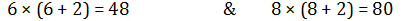# Reasoning Advanced level Quiz For SSC CGL : 15th Jan. 2020 for analogy and figure counting

Q1. From the given figures, which figure is the correct water image of the question figure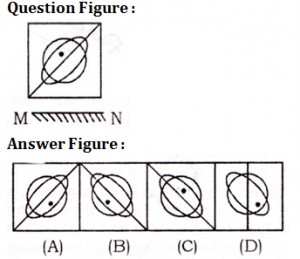(a) A
(b) B
(c) C
(d) D

Q2. Folding a square piece of paper, they have been cut according to the below depicted figures. How will it took like on opening ?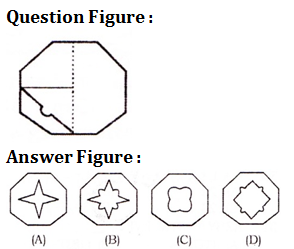(a) A
(b) B
(c) C
(d) D

Q3. How many triangles are there in the given figure ?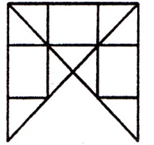(a) 14
(b) 16 or more
(c) 13
(d) 12

Q4. In the given figure, circle stands for intelligent, square for hard-working, triangle for post-graduate and the rectangle for loyal employees. Study the figure and indicate the number which represents hard-working post-graduate employees who are neither intelligent nor loyal.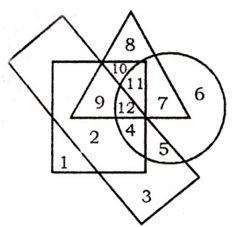(a) 5
(b) 4
(c) 10
(d) 9

Directions (5-10): Select the related word/ letters/ number/figure from the given alternatives.

Q5.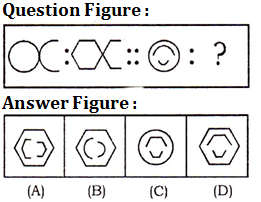(a) A
(b) B
(c) C
(d) D

Q6. Memory : Amnesia : : Movement : ?
(a) Meningitis
(b) Cirrhosis
(c) Aphasia
(d) Paralysis

Q7. Square : Cube : : Triangle : ?
(a) Circle
(b) Tetrahedron
(c) Cube
(d) Cuboid

Q8. DAER : TLEM : : HEIV : ?
(a) ALTE
(b) XPIQ
(c) KTEI
(d) OMRE

Q9. DARING : FCTKPI : : BRIGHT : ?
(a) DTKIJV
(b) CTKIJV
(c) DTJIHV
(d) DSLHJV

Q10. 6 : 48 : 8 : ?
(a) 90
(b) 80
(c) 63
(d) 78

Solutions:

S1. Ans.(b)

S2. Ans.(d)

S3. Ans.(b)
Sol.
There are 20 triangle.

S4. Ans.(c)

S5. Ans.(d)

S6. Ans.(d)
Sol.
Paralysis is related to movement

S7. Ans.(b)
Sol.
Tetrahedron is formed by triangle

S8. Ans(b)
Sol.
The pattern between 1st and 3rd is +4, +4, +4, +4 same relation is between 2nd and 4th.

S9. Ans.(a)
Sol.
Each letter of 1st term is moved two steps to obtain corresponding letter of 2nd term similarly 4th is obtained from 3rd.

S10. Ans.(b)
Sol.